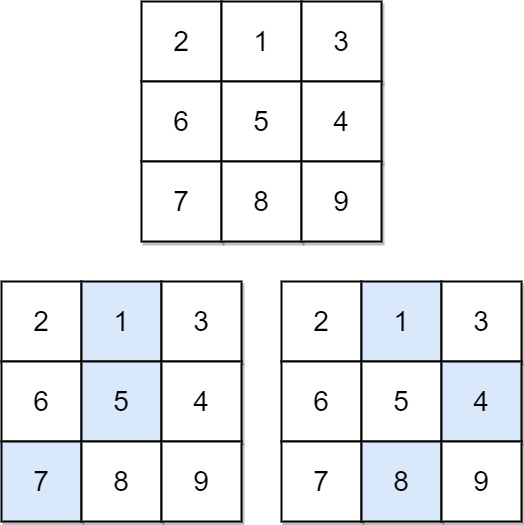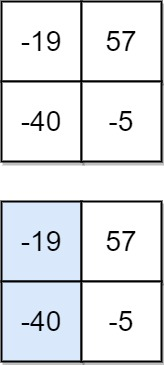931. Minimum Falling Path Sum
Medium
4.6K
120

Given an `n x n` array of integers `matrix`, return the minimum sum of any falling path through `matrix`.

A falling path starts at any element in the first row and chooses the element in the next row that is either directly below or diagonally left/right. Specifically, the next element from position `(row, col)` will be `(row + 1, col - 1)`, `(row + 1, col)`, or `(row + 1, col + 1)`.

Example 1:```Input: matrix = [[2,1,3],[6,5,4],[7,8,9]]
Output: 13
Explanation: There are two falling paths with a minimum sum as shown.
```

Example 2:```Input: matrix = [[-19,57],[-40,-5]]
Output: -59
Explanation: The falling path with a minimum sum is shown.
```

Constraints:

• `n == matrix.length == matrix[i].length`
• `1 <= n <= 100`
• `-100 <= matrix[i][j] <= 100`
Accepted
237.5K
Submissions
343.5K
Acceptance Rate
69.1%

Seen this question in a real interview before?
1/4
Yes
No

Discussion (0)

Related Topics